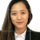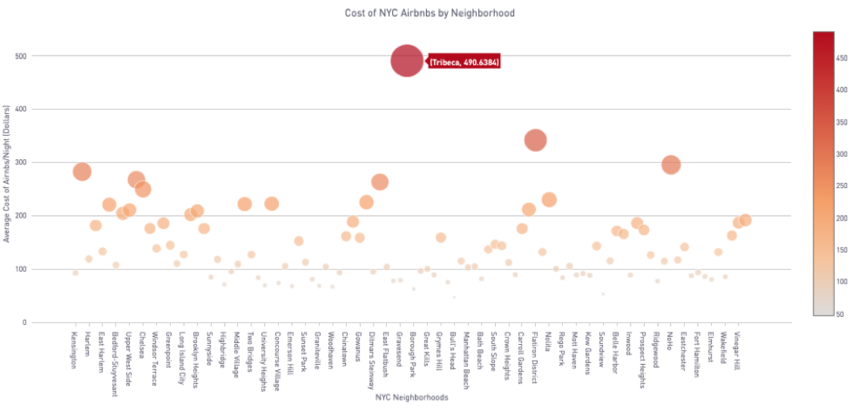# Setting the Marker/Bubble Size in Bubble ChartsBackground

One of the more frequent questions that we get asked on Periscope is how a user can alter/set the size of the bubbles/markers beyond the default “weight options” as seen in some of our built-in bubble chart templates  (i.e. map charts).

The answer for creating custom bubbles/markers sizes is setting the size of these markers/bubbles through our Plot.ly integration. For this analysis, we will be using a dataset that describes the listing activity and metrics for Airbnbs in New York City (see attached file).

For this case study, our SQL output returns the following two columns:

• Neighborhood: The Airbnb’s geographic neighborhood in New York City
• Average_price: The average price of an Airbnb in that neighborhood

Setting the Bubble Size

In this line of Python code, we are setting the x values to the "Neighborhood" column, y values to "Average_Price" column, and setting the chart's mode to markers (which simply means that we want the data to manifest as bubbles/scatter points).

```data = [go.Scatter(x=df['neighborhood'], y=df['average_price'],mode='markers',marker=dict(size=0.1*df['average_price’],color=df['average_price’],showscale=True))]
```

To set the size of the markers, we will have to create a dictionary:

`marker=dict(size=df['average_price']...`

Another interesting aspect of the Plot.ly library is its flexibility in adjusting the size of the markers. In this case, the objective was to correlate the size of the bubbles to the average price of the Airbnbs. However, due to the rather large size of the markers, I decided to multiply the values in the "Average_Price" column by 0.1 to reduce their overall size.

`marker=dict(size=0.1*df['average_price']...`

Then, we set the colors of the bubbles to the RGB equivalents of their values, as well as include the color scale for reference.

`marker=dict(size=0.1*df['average_price’],color=df['average_price’],showscale=True))]`

Ta-da! Now, we have a bubble chart with custom sizing!

Code

This bare-bones Python 3.7 code is inspired by Plot.ly's documentation,  and it creates the bubble chart as seen above in this post:

```#Objective: Create a bubble chart where we can have the ability to set the size of the bubbles
#Dataset: This dataset from Kaggle describes the listing activity and metrics in NYC, NY for 2019

#Columns:
# Primary Key, Numeric: ID --'listing id'
# String: Name --'name of the listing'
# Foreign Key, Numeric: host_id --'host id'
# String: host_name --'name of the host'
# String: neighbourhood_group --'location'
# String: neighbourhood --'area'
# Latitude Coordinates: latitude --'latitude coordinates'
# Longitude Coordinates: longitude --'longitude coordinates'
# String: room_type --'listing space type'
# Numeric: price --'price in dollars'
# Numeric: minimum_nights --'amount of nights minimum'
# Numeric: number_of_reviews --'number of reviews'
# Datetime: last_review --'latest review'
# Numeric: reviews_per_month --'number of reviews per month'
# Numeric: calculated_host_listings_count --'amount of listing per host'
# Numeric: availability_365 --'number of days when listing is avaliable for booking'

#SQL output is imported as a dataframe variable called 'df'
import pandas as pd
import plotly.plotly as py
import plotly.graph_objs as go

#This line shows the periscope data frame, and is primarily used to just visualize the SQL output.
periscope.table(df)

#Next, we are going to create the data points.
data=[go.Scatter(
x=df['neighborhood'], y=df['average_price'],mode='markers', marker=dict(size=0.1*df['average_price'],color=df['average_price'],showscale=True))]

#Then we will want to label the chart's title, x-axis, y-axis, and also set the margins of the chart.
layout = go.Layout(title='Cost of NYC Airbnbs by Neighborhood', xaxis = {'title':'NYC Neighborhoods'}, yaxis = dict(title = 'Average Cost of Airnbs/Night (Dollars)'), margin=dict(l=50,r=50,b=125,t=25),hovermode='closest')

#Let's create the layout and plot out the data points.
fig = go.Figure(data=data,layout=layout)

#Now, we can visualize the final figure.
periscope.plotly(fig)
```

If you are interested in further customizations such as setting the markers to different colors or alternative layouts, please browse around the plot.ly figure reference guide.